# Quick Start guide¶

## Hello, world!¶

To get started, let’s simulate a basic model and plot it. For simplicity, we will use all of the model defaults.

```import matplotlib.pyplot as plt
from pyddm import Model
m = Model()
s = m.solve()
plt.plot(s.t_domain, s.pdf("correct"))
plt.savefig("helloworld.png")
plt.show()
```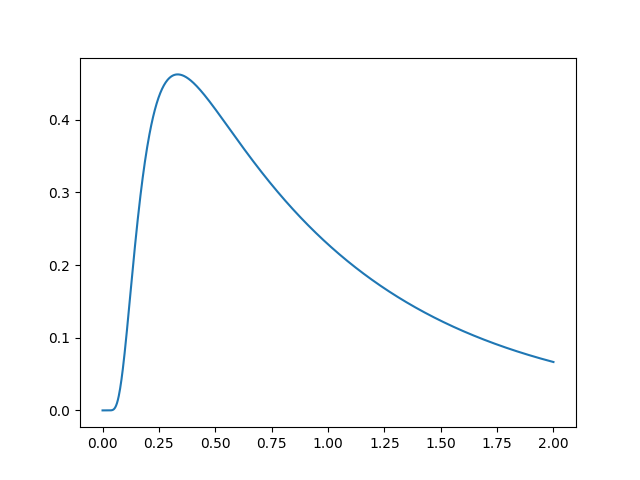Congratulations! You’ve successfully simulated your first model! Let’s dig in a bit so that we can define more useful models.

`Download this full example`

## Simple example¶

The following simulates a simple DDM with constant drift. First, it shows how to build a model and then uses it to generate artificial data. After the artificial data has been generated, it fits a new model to these data and shows that the parameters are similar.

`Model` is the object which represents a DDM. Its default behavior can be changed through `Drift`, `Noise`, `Bound`, `InitialCondition`, and `Overlay` objects, which specify the behavior of each of these model components. Each model must have one of each of these, but defaults to a simple case for each. These determine how it calculates the drift rate (`Drift`), diffusion coefficient (`Noise`), shape of the integration boundaries (`Bound`), initial particle distribution (`InitialCondition`), and any other modifications to the generated solution (`Overlay`).

Each of these model components may take “parameters” which are (usually) unique to that specific model component. For instance, the `DriftConstant` class takes the “drift” parameter, which should be passed to the constructor. Similarly, `NoiseConstant` takes the parameter “noise” to determine the standard deviation of the drift process, and `OverlayNonDecision` takes “nondectime”, the non-decision time (efferent/afferent/apparatus delay) in seconds. Some model components, such as `ICPointSourceCenter` which represents a starting point initial condition directly in between the bounds, does not take any parameters.

For example, the following is a DDM with drift 2.2, noise 1.5, bound 1.1, and a 100ms non-decision time. It is simulated for 2 seconds (`T_dur`) with reasonable timestep and grid size (`dt` and `dx`). Once we define the model, the `solve()` function runs the simulation. This model can be described as shown below:

```from pyddm import Model
from pyddm.models import DriftConstant, NoiseConstant, BoundConstant, OverlayNonDecision, ICPointSourceCenter

model = Model(name='Simple model',
drift=DriftConstant(drift=2.2),
noise=NoiseConstant(noise=1.5),
bound=BoundConstant(B=1.1),
overlay=OverlayNonDecision(nondectime=.1),
dx=.001, dt=.01, T_dur=2)
display_model(model)
sol = model.solve()
```

Solution objects represent the probability distribution functions over time for choices associated with upper and lower bound crossings. By default, this is “correct” and “error” responses, respectively. We can generate psuedo-data from this solved model with the `resample()` function:

```samp = sol.resample(1000)
```

To fit the outputs, we first create a model with special `Fittable` objects in all the parameters we would like to fit. We specify the range of each of these objects as a hint to the optimizer; this is mandatory for some but not all optimization methods. Then, we run the `fit_adjust_model()` function, which will convert the `Fittable` objects to `Fitted` objects and find a value for each which collectively minimizes the objective function.

Here, we use the same model as above, since we know the form the model is supposed to have. We fit the model to the generated data using BIC as a loss function and differential evolution to optimize the parameters:

```from pyddm import Fittable, Fitted
from pyddm.models import LossRobustBIC
model_fit = Model(name='Simple model (fitted)',
drift=DriftConstant(drift=Fittable(minval=0, maxval=4)),
noise=NoiseConstant(noise=Fittable(minval=.5, maxval=4)),
bound=BoundConstant(B=1.1),
overlay=OverlayNonDecision(nondectime=Fittable(minval=0, maxval=1)),
dx=.001, dt=.01, T_dur=2)

fitting_method="differential_evolution",
lossfunction=LossRobustBIC, verbose=False)
```

We can display the newly-fit parameters with the `display_model()` function:

```display_model(model_fit)
```

This shows that the fitted value of drift is 2.2096, which is close to the value of 2.2 we used to simulate it. Similarly, noise fits to 1.539 (compared to 1.5) and nondectime (non-decision time) to 0.1193 (compared to 0.1). The fitting algorithm is stochastic, so exact values may vary slightly:

```Model Simple model (fitted) information:
Drift component DriftConstant:
constant
Fitted parameters:
- drift: 2.209644
Noise component NoiseConstant:
constant
Fitted parameters:
- noise: 1.538976
Bound component BoundConstant:
constant
Fixed parameters:
- B: 1.100000
IC component ICPointSourceCenter:
point_source_center
(No parameters)
Overlay component OverlayNonDecision:
Add a non-decision by shifting the histogram
Fitted parameters:
- nondectime: 0.119300
Fit information:
Loss function: BIC
Loss function value: 562.1805934500456
Fitting method: differential_evolution
Solver: auto
Other properties:
- nparams: 3
- samplesize: 1000
- mess: ''
```

While the values are close to the true values, the fit is not perfect due to the finite size of the resampled data. If we want to use these programmatically, we can use the `parameters()` function, like:

```model_fit.parameters()
```

The `Fitted` objects it returns can be used anywhere as if it is a normal number/float. (Actually, `Fitted` is a subclass of “float”!)

We can also examine different properties of the fitting process in the `FitResult` object. For instance, to get the value of the loss function, we can do:

```model_fit.get_fit_result().value()
```

We can also draw a plot visualizing the fit. Unlike our first example, we will now use one of PyDDM’s convenience methods, `plot_fit_diagnostics()`:

```import pyddm.plot
import matplotlib.pyplot as plt
pyddm.plot.plot_fit_diagnostics(model=model_fit, sample=samp)
plt.savefig("simple-fit.png")
plt.show()
```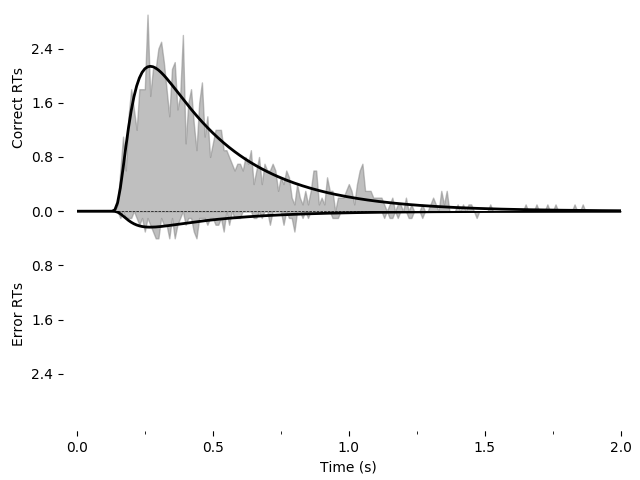Using the `Solution` object `sol` we have access to a number of other useful functions. For instance, we can find the probability of a response using `prob()`, such as `sol.prob("correct")` for the probability of a correct response, or the entire histogram of responses using `pdf()`, such as `sol.pdf("error")` for the distribution of errors.

```print(sol.prob("correct"))
print(sol.pdf("error"))
```

See `the Solution object documentation` for more such functions.

We could also named the upper and lower boundary as something else (e.g. “left” and “right” response), sometimes called “stimulus coding”. To do this, we need to pass the “choice_names” parameter to the Sample and the Model object. See the section on stimulus coding

To save the model for later use, we can use the repr() function built-in to Python. This function outputs a string with all the information needed to recreate the object. It is very similar to the print() function, except it saves the result as a string rather than displaying it. To save, do the following:

```with open("model.txt", "w") as f:
f.write(repr(model_fit))
```

Then, you can load the saved model with the following. You may need to add additional imports from PyDDM if you get an “import error”.

```from pyddm import FitResult
with open("model.txt", "r") as f:
```

`Download this full example`

## Working with data¶

In this example, we load data from the open dataset by Roitman and Shadlen (2002). This dataset can be downloaded here and the relevant data extracted `with our script`. The processed CSV file can be `downloaded directly`.

The CSV file generated from this looks like the following:

monkey rt coh correct trgchoice
1 0.355 0.512 1.0 2.0
1 0.359 0.256 1.0 1.0
1 0.525 0.128 1.0 1.0

It is fairly easy then to load and process the CSV file:

```from pyddm import Sample
import pandas
with open("roitman_rts.csv", "r") as f:

df_rt = df_rt[df_rt["monkey"] == 1] # Only monkey 1

# Remove short and long RTs, as in 10.1523/JNEUROSCI.4684-04.2005.
# This is not strictly necessary, but is performed here for
# compatibility with this study.
df_rt = df_rt[df_rt["rt"] > .1] # Remove trials less than 100ms
df_rt = df_rt[df_rt["rt"] < 1.65] # Remove trials greater than 1650ms

# Create a sample object from our data.  This is the standard input
# format for fitting procedures.  Since RT and correct/error are
# both mandatory columns, their names are specified by command line
# arguments.
roitman_sample = Sample.from_pandas_dataframe(df_rt, rt_column_name="rt", choice_column_name="correct")
```

This gives an output sample with the conditions “monkey”, “coh”, and “trgchoice”.

Note that this examples requires pandas to be installed.

```from pyddm import Sample
import numpy as np
with open("roitman_rts.csv", "r") as f:

# RT data must be the first column and correct/error must be the
# second column.
rt = M[:,1].copy() # Use .copy() because np returns a view
corr = M[:,3].copy()
monkey = M[:,0].copy()
M[:,0] = rt
M[:,1] = corr
M[:,3] = monkey

# Only monkey 1
M = M[M[:,3]==1,:]

# As before, remove longest and shortest RTs
M = M[M[:,0]>.1,:]
M = M[M[:,0]<1.65,:]

conditions = ["coh", "monkey", "trgchoice"]
roitman_sample2 = Sample.from_numpy_array(M, conditions)
```

We can confirm that these two methods of loading data produce the same results:

```assert roitman_sample == roitman_sample2
```

### Fitting a model to data¶

Now that we have loaded these data, we can fit a model to them. First we will fit a DDM, and then we will fit a GDDM.

First, we want to let the drift rate vary with the coherence. To do so, we must subclass `Drift`. Each subclass must contain a name (a short description of how drift varies), required parameters (a list of the parameters that must be passed when we initialize our subclass, i.e. parameters which are passed to the constructor), and required conditions (a list of conditions that must be present in any data when we fit data to the model). We can easily define a model that fits our needs:

```import pyddm as ddm
class DriftCoherence(ddm.models.Drift):
name = "Drift depends linearly on coherence"
required_parameters = ["driftcoh"] # <-- Parameters we want to include in the model
required_conditions = ["coh"] # <-- Task parameters ("conditions"). Should be the same name as in the sample.

# We must always define the get_drift function, which is used to compute the instantaneous value of drift.
def get_drift(self, conditions, **kwargs):
return self.driftcoh * conditions['coh']
```

Because we are fitting with likelihood, we must include a baseline lapse rate to avoid taking the log of 0. Traditionally this is implemented with a uniform distribution, but PyDDM can also use an exponential distribution using `OverlayPoissonMixture` (representing a Poisson process lapse rate), as we use here. However, since we also want a non-decision time, we need to use two Overlay objects. To accomplish this, we can use an `OverlayChain` object. Then, we can construct a model which uses this and fit the data to the model:

```from pyddm import Model, Fittable
from pyddm.models import NoiseConstant, BoundConstant, OverlayChain, OverlayNonDecision, OverlayPoissonMixture
model_rs = Model(name='Roitman data, drift varies with coherence',
drift=DriftCoherence(driftcoh=Fittable(minval=0, maxval=20)),
noise=NoiseConstant(noise=1),
bound=BoundConstant(B=Fittable(minval=.1, maxval=1.5)),
# Since we can only have one overlay, we use
# OverlayChain to string together multiple overlays.
# They are applied sequentially in order.  OverlayNonDecision
# implements a non-decision time by shifting the
# resulting distribution of response times by
# `nondectime` seconds.
overlay=OverlayChain(overlays=[OverlayNonDecision(nondectime=Fittable(minval=0, maxval=.4)),
OverlayPoissonMixture(pmixturecoef=.02,
rate=1)]),
dx=.001, dt=.01, T_dur=2)

# Fitting this will also be fast because PyDDM can automatically
# determine that DriftCoherence will allow an analytical solution.
```

Finally, we can display the fit parameters with the following command:

```display_model(fit_model_rs)
```

This gives the following output (which may vary slightly, since the fitting algorithm is stochastic):

```Model Roitman data, drift varies with coherence information:
Drift component DriftCoherence:
Drift depends linearly on coherence
Fitted parameters:
- driftcoh: 10.388292
Noise component NoiseConstant:
constant
Fixed parameters:
- noise: 1.000000
Bound component BoundConstant:
constant
Fitted parameters:
- B: 0.744209
IC component ICPointSourceCenter:
point_source_center
(No parameters)
Overlay component OverlayChain:
Overlay component OverlayNonDecision:
Add a non-decision by shifting the histogram
Fitted parameters:
- nondectime: 0.312433
Overlay component OverlayPoissonMixture:
Poisson distribution mixture model (lapse rate)
Fixed parameters:
- pmixturecoef: 0.020000
- rate: 1.000000
Fit information:
Loss function: Negative log likelihood
Loss function value: 199.3406727870083
Fitting method: differential_evolution
Solver: auto
Other properties:
- nparams: 3
- samplesize: 2611
- mess: ''
```

Or, to access them within Python instead of printing them,

```fit_model_rs.parameters()
```

Note that if you see “Warning: renormalizing model solution from X to 1.” for some X, this is okay as long as X is close (or so) to 1.0 or as long as this is seen early in the fitting procedure. If it is larger or seen towards the end of the fitting procedure, consider using smaller dx or dt in the simulation. This indicates numerical imprecision in the simulation.

### Plotting the fit¶

We can also graphically evaluate the quality of the fit. We can plot and save a graph:

```import pyddm.plot
import matplotlib.pyplot as plt
pyddm.plot.plot_fit_diagnostics(model=fit_model_rs, sample=roitman_sample)
plt.savefig("roitman-fit.png")
plt.show()
```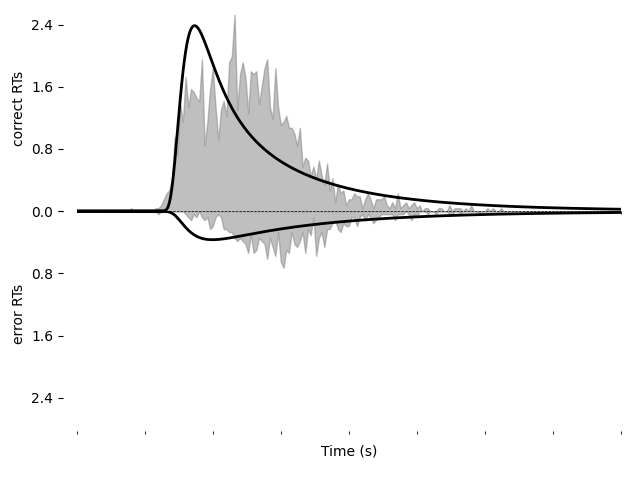This model does not seem to fit the data very well.

We can alternatively explore this with the PyDDM’s model GUI:

```pyddm.plot.model_gui(model=fit_model_rs, sample=roitman_sample)
```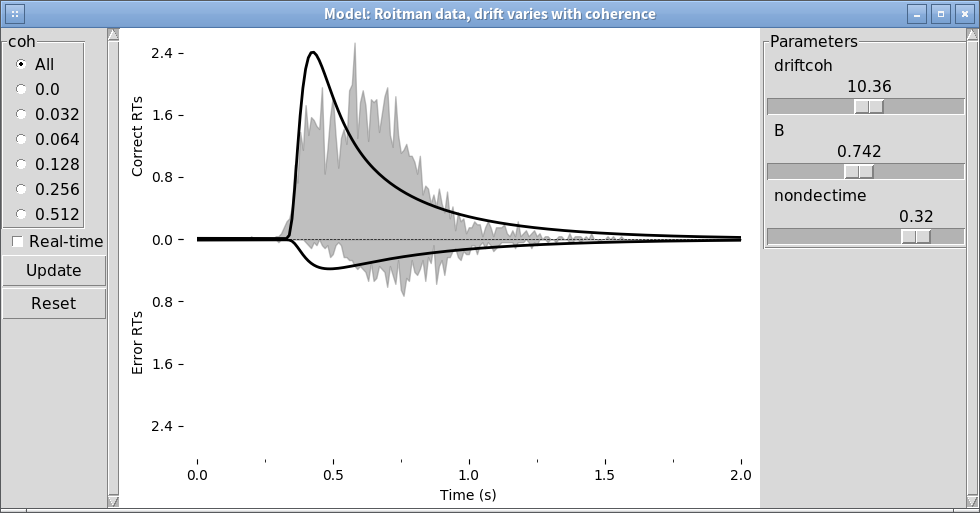`Download this full example`

## Improving the fit¶

Let’s see if we can improve the fit by including additional model components. We will include exponentially collapsing bounds and use a leaky or unstable integrator instead of a perfect integrator.

To use a coherence-dependent leaky or unstable integrator, we can build a drift model which incorporates the position of the decision variable to either increase or decrease drift rate. This can be accomplished by making `get_drift` depend on the argument `x`.

```class DriftCoherenceLeak(ddm.models.Drift):
name = "Leaky drift depends linearly on coherence"
required_parameters = ["driftcoh", "leak"] # <-- Parameters we want to include in the model
required_conditions = ["coh"] # <-- Task parameters ("conditions"). Should be the same name as in the sample.

# We must always define the get_drift function, which is used to compute the instantaneous value of drift.
def get_drift(self, x, conditions, **kwargs):
return self.driftcoh * conditions['coh'] + self.leak * x
```

Collapsing bounds are already included in PyDDM, and can be accessed with `BoundCollapsingExponential`.

Thus, the full model definition is

```from pyddm.models import BoundCollapsingExponential
model_leak = Model(name='Roitman data, leaky drift varies with coherence',
drift=DriftCoherenceLeak(driftcoh=Fittable(minval=0, maxval=20),
leak=Fittable(minval=-10, maxval=10)),
noise=NoiseConstant(noise=1),
bound=BoundCollapsingExponential(B=Fittable(minval=0.5, maxval=3),
tau=Fittable(minval=.0001, maxval=5)),
# Since we can only have one overlay, we use
# OverlayChain to string together multiple overlays.
# They are applied sequentially in order.  OverlayDelay
# implements a non-decision time by shifting the
# resulting distribution of response times by
# `delaytime` seconds.
overlay=OverlayChain(overlays=[OverlayNonDecision(nondectime=Fittable(minval=0, maxval=.4)),
OverlayPoissonMixture(pmixturecoef=.02,
rate=1)]),
dx=.01, dt=.01, T_dur=2)
```

Before fitting this model, let’s look at it in the model GUI:

```from pyddm.plot import model_gui
model_gui(model_leak, sample=roitman_sample)
```

We can fit this and save it as an image using the following. Note that this may take a while (hours) due to the increased number of parameters and because the earlier examples were able to use the analytical solver but the present example must use backward Euler. For all coherences, the fit is:

```fit_model_leak = fit_adjust_model(sample=roitman_sample, model=model_leak, verbose=False)
pyddm.plot.plot_fit_diagnostics(model=fit_model_leak, sample=roitman_sample)
plt.savefig("leak-collapse-fit.png")
```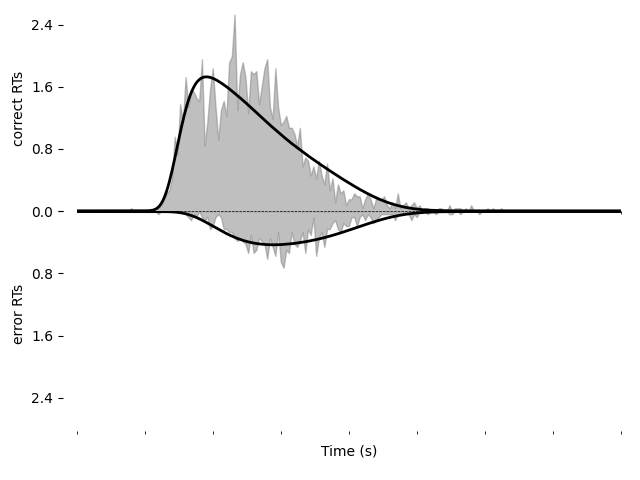## Going further¶

Just as we created DriftCoherence above (by inheriting from `Drift`) to modify the drift rate based on coherence, we can modify other portions of the model. See PyDDM Cookbook for more examples. Also see the API Documentation for more specific details about overloading classes.

## Summary¶

PyDDM can simulate models and generate artificial data, or it can fit them to data. Below are high-level overviews for how to accomplish each.

To simulate models and generate artificial data:

1. Optionally, define unique components of your model. Models are modular, and allow specifying a dynamic drift rate, noise level, diffusion bounds, starting position of the integrator, or post-simulation modifications to the RT histogram. Many common models for these are included by default, but for advance functionality you may need to subclass `Drift`, `Noise`, `Bound`, `InitialCondition`, or `Overlay`. These model components may depend on “conditions”, i.e. prespecified values associated with the behavioral task which change from trial to trial (e.g. stimulus coherence), or “parameters”, i.e. values which apply to all trials and should be fit to the subject.
2. Define a model. Models are represented by creating an instance of the `Model` class, and specifying the model components to use for it. These model component can either be the model components included in PyDDM or ones you created in step 1. Values must be specified for all parameters required by model components.
3. Simulate the model using the `Model.solve()` method to generate a `Solution` object. If you have multiple conditions, you must run `Model.solve()` separately for each set of conditions and generate separate `Solution` objects.
4. Run the `Solution.resample()` method of the `Solution` object to generate a `Sample`. If you have multiple `Solution` objects (for multiple task conditions), you will need to generate multiple `Sample` objects as well. These can be added together with the “+” operator to form one single `Sample` object.

To fit a model to data:

1. Optionally define unique components of your model, as mentioned in Step 1 above.
2. Load your data into a `Sample` object using either `Sample.from_numpy_array()` or `Sample.from_pandas_dataframe()`. If you have multiple task conditions (i.e. prespecified values associated with the behavioral task which change from trial to trial), make sure that the names of the conditions in the `Sample` object align with those that are used in your model components. (In other words, if a model component expects a coherence value named “coh”, make sure your sample includes a coherence value named “coh”.)
3. Define a model. Models are represented by creating an instance of the `Model` class, and specifying the model components to use for it. These model component can either be the model components included in PyDDM or ones you created in step 1. Parameters for the model components must either be specified expicitly or else set to a `Fittable` instance, for example “Fittable(minval=0, maxval=1)”.
1. Run `fit_adjust_model()` on the model and the sample. Optionally specify a `loss function` other than the default (which uses BIC). After fitting, the `Fittable` objects in the model will be changed to `Fitted` objects, which are just like `Fittable` objects except they contain the fitted values.
2. View the output by calling `display_model()` on the model. The value of the loss function is accessible via `Model.get_fit_result()` and the parameters via `Model.parameters()`.